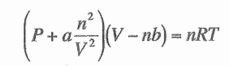# Problem: Use the van der Waal's equation to calculate the pressure (in atm) exerted by 1.00 mol of chlorine gas confined to a volume of 2.00 L at 273K. The value of a = 6.49 L2 atm mol-2, and that of b = 0.0562 L mol-1 for chlorine gas.a) no given answer is closeb) 9.9c) 4.12d) 1.54e) 3.73

###### FREE Expert Solution
90% (127 ratings)
###### FREE Expert Solution

We’re being asked to calculate the pressure exerted by a chlorine gas using the Van der Waal’s equation

The Van der Waals equation is shown below:

$\overline{)\left(\mathbf{P}\mathbf{+}\mathbf{a}\frac{{\mathbf{n}}^{\mathbf{2}}}{{\mathbf{V}}^{\mathbf{2}}}\right)\left(\mathbf{V}\mathbf{-}\mathbf{n}\mathbf{b}\right){\mathbf{=}}{\mathbf{n}}{\mathbf{R}}{\mathbf{T}}}$

P = pressure, atm
V = volume, L
n = # of moles, mol
R = gas constant = 0.08206 (Latm)/(molK)
T = temperature, K
a = polarity coefficient
= size coefficient

Let’s first isolate the pressure in the Van der Waals Equation:

90% (127 ratings)###### Problem Details

Use the van der Waal's equation to calculate the pressure (in atm) exerted by 1.00 mol of chlorine gas confined to a volume of 2.00 L at 273K. The value of a = 6.49 L2 atm mol-2, and that of b = 0.0562 L mol-1 for chlorine gas.

a) no given answer is close

b) 9.9

c) 4.12

d) 1.54

e) 3.73What scientific concept do you need to know in order to solve this problem?

Our tutors have indicated that to solve this problem you will need to apply the Van der Waals Equation concept. You can view video lessons to learn Van der Waals Equation. Or if you need more Van der Waals Equation practice, you can also practice Van der Waals Equation practice problems.

What is the difficulty of this problem?

Our tutors rated the difficulty ofUse the van der Waal's equation to calculate the pressure (i...as high difficulty.

How long does this problem take to solve?

Our expert Chemistry tutor, Dasha took 6 minutes and 36 seconds to solve this problem. You can follow their steps in the video explanation above.

What professor is this problem relevant for?

Based on our data, we think this problem is relevant for Professor Mammana's class at UDAYTON.